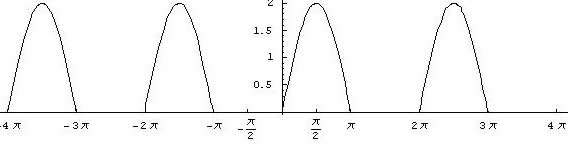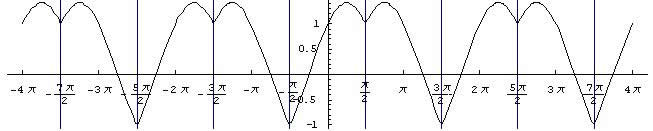#### You may also like### Degree Ceremony

What does Pythagoras' Theorem tell you about these angles: 90°, (45+x)° and (45-x)° in a triangle?### After Thought

Which is larger cos(sin x) or sin(cos x) ? Does this depend on x ?### Small Steps

Two problems about infinite processes where smaller and smaller steps are taken and you have to discover what happens in the limit.

# Loch Ness

##### Age 16 to 18Challenge Level

Congratulations to Andrei from Tudor Vianu National College, Bucharest, Romania for this excellent solution.

(1) Plot the graph of the function $y=f(x)$ where $f(x) = \sin x +|\sin x|$. Differentiate the function and say where the derivative is defined and where it is not defined.

I observe that $\sin x$ takes positive values between $2k\pi$ and $(2k+1)\pi$ (for integer $k$), that is in the first two quadrants, and $\sin x$ takes negative values between $(2k+1)\pi$ and $(2k+2)\pi$, that is in the third and fourth quadrants. So

\eqalign{ f(x) &= 2\sin x \quad for\ 2k\pi \leq x \leq (2k+1)\pi \cr &= 0 \quad for \ (2k+1)\pi \leq x \leq (2k+2)\pi .}

Below is represented the graph of $y = f(x)$:Now I calculate the first derivative of $f(x)$:

\eqalign{ f^\prime (x) &= 2\cos x \quad for\ 2k\pi < x < (2k+1)\pi \cr &= 0 \quad for\ (2k+1)\pi < x < (2k+2)\pi}

The derivative $f^\prime(x)$ is not defined at the points $x = k\pi$ for any integer $k$ and it does not have a tangent at these points.

(2) Now I express the function $f(x) = \sin x + \cos x$ in the form $f(x)=A\sin (x+\alpha)$, find $A$ and $\alpha$ and plot the graph of this function. Similarly I express the function $g(x) = \sin x - \cos x$ in the form $g(x) = B\sin (x +\beta)$ where $-\pi /2 < \beta < \pi /2$, and plot its graph on the same axes.

\eqalign { f(x) &= \sin x + \cos x = A\sin (x + \alpha) \cr &= A\sin x\cos\alpha + A\cos x \sin \alpha }

As this formula must be valid for any $x$, I obtain: $$A\sin \alpha = 1 \quad {\rm and} \ A\cos \alpha = 1$$ and hence $\tan \alpha = 1$, $\alpha = \pi/4$ and $A=\pm \sqrt 2$.

Comment: If $A$ has the meaning of an amplitude, $A$ is positive, and only the positive solution must be kept. This type of problem is typical for the composition of oscillations.

Hence the graph of this function is a sine graph with a phase shift of $\pi/4$, that is $f(x)=0$ when $x=k\pi - \pi/4$, it takes the value 1 when $x=2k\pi$ and $x=2k\pi + \pi/2$ and the value -1 when $x=(2k+1)\pi$ and $x=2k\pi - \pi/2$, has maximum values $(2k\pi +\pi/4, \sqrt 2)$ and minimum values $((2k+1)\pi + \pi/4, -\sqrt 2)$

In a similar manner I write $g(x) = \sin x - \cos x$:

\eqalign { f(x) &= \sin x - \cos x = B\sin (x + \beta) \cr &= B\sin x\cos\beta + B\cos x \sin \beta }

So I have $$B\sin \beta = -1 \quad {\rm and} \ B\cos \beta = 1$$ and hence $\tan \beta = -1$, $\beta = -\pi/4$ and $B=\sqrt 2$. Hence the graph of this function is a sine graph with a phase shift of $-\pi/4$, that is $f(x)=0$ when $x=k\pi + \pi/4$, it takes the value 1 when $x=k\pi$ and $x=2k\pi + \pi/2$, has maximum values $(2k+1)\pi - \pi/4, \sqrt 2)$ and minimum values $(2k\pi - \pi/4, -\sqrt 2)$.(3) Now, I calculate the function $f(x) = \sin x + |\cos x|$ and plot the graph.

\eqalign{ f(x) &= \sin x + \cos x = \sqrt 2 \sin(x + \pi /4) \ for \ 2k\pi -\pi/2\leq x \leq 2k\pi +\pi/2 \cr &= \sin x - \cos x = \sqrt 2 \sin(x - \pi /4)\ for \ 2k\pi + \pi/2 \leq x \leq 2k\pi + 3\pi/2 .}

The derivative is not defined at $x= k\pi + \pi/2$ and for other values of $x$ it is:

\eqalign{ f^\prime(x) &= \cos x - \sin x \ {\rm for} \ 2k\pi -\pi/2< x < 2k\pi +\pi/2 \cr &= \cos x + \sin x \ {\rm for} \ 2k\pi + \pi/2 < x < 2k\pi + 3\pi/2 .}